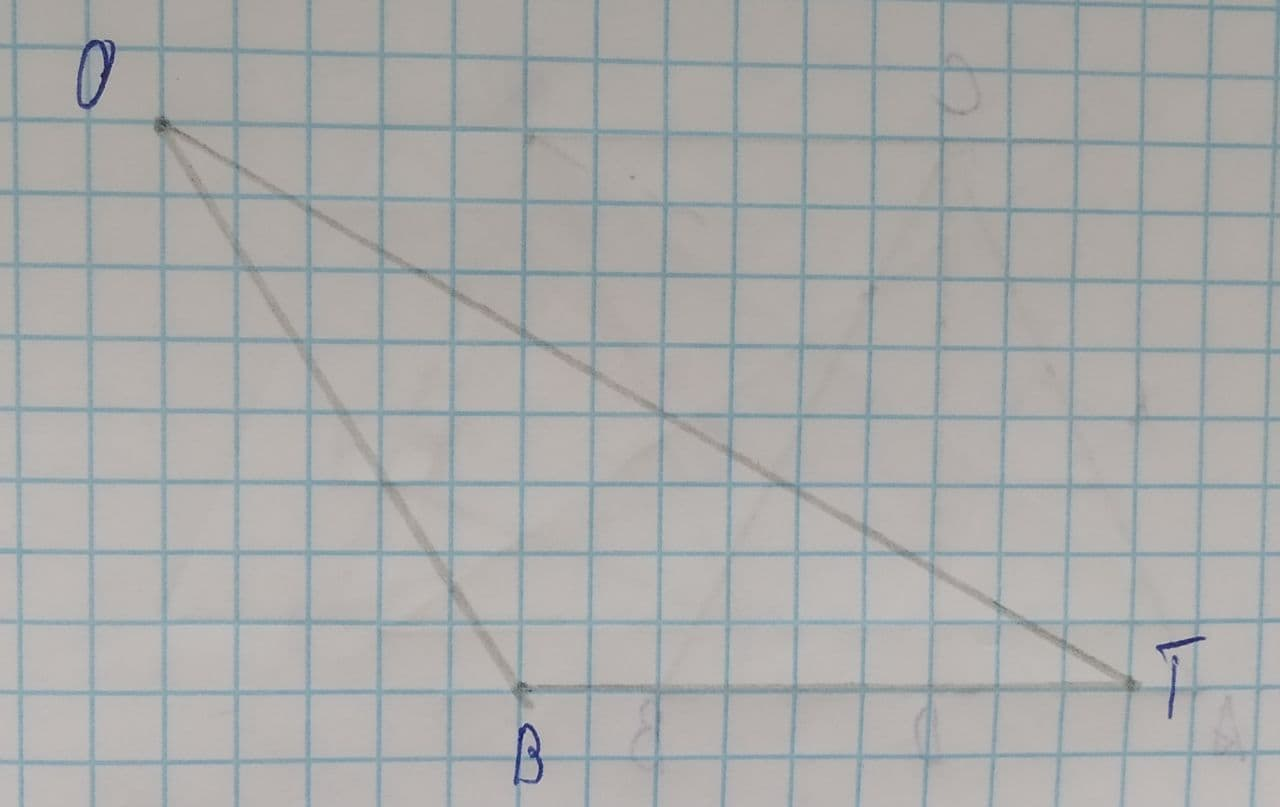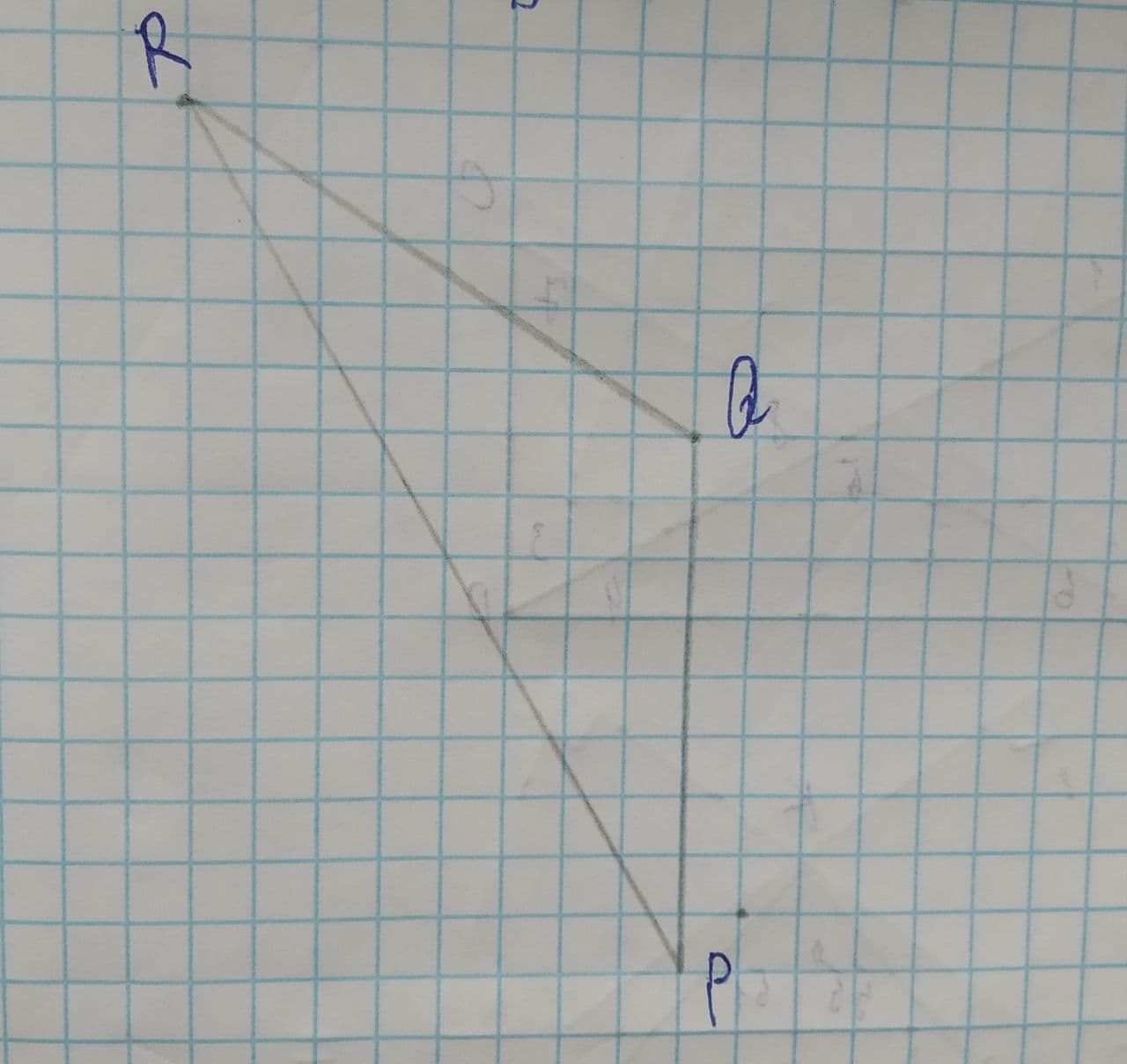# Explain how to construct a triangle that is congruent to \triangle OBTpostillan4 2021-07-13 Answered
To explain: How to construct a triangle that is congruent to $\mathrm{△}OBT$.You can still ask an expert for help

## Want to know more about Congruence?

• Questions are typically answered in as fast as 30 minutes

Solve your problem for the price of one coffee

• Math expert for every subject
• Pay only if we can solve itLuvottoq

Here, $\mathrm{△}OBT$ is an obtuse triangle as $\mathrm{\angle }OBT>{90}^{\circ }$.
According to SSS congruence criteria, if all the sides of one triangle are congruent to the corresponding sides of other triangle then the two triangles are said to be congruent.
Construct $\mathrm{△}PQR$ such that $PQ\stackrel{\sim }{=}OB,PR\stackrel{\sim }{=}OT,QR\stackrel{\sim }{=}BT$.
So,
$\mathrm{△}OBT\stackrel{\sim }{=}\mathrm{△}PQR$ (by SSS congruence criteria)